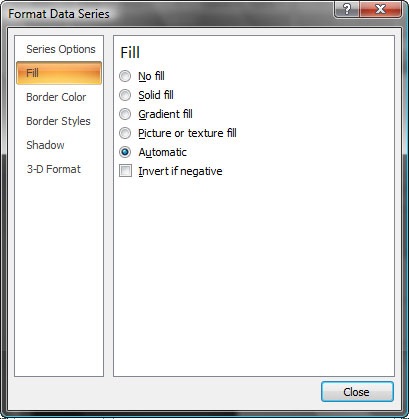# Charts in PowerPoint, Using the Invert if Negative Option in PowerPoint 2010 and 2007 for Windows

Highlight negative values in a chart by using the Invert if Negative option in PowerPoint 2010 and 2007 for Windows.

Author:

Product/Version: PowerPoint 2010 and 2007 for Windows

OS: Windows XP and higher

If your chart has negative value PowerPoint by default uses the same fill color for negative values in any chart (see Figure 1) -- you can anyhow override that with a convenient Invert if Negative option that we explored towards the end of the Changing Fills and Outlines of Charts tutorial.Figure 1: Chart with negative figures

Note: The Invert if Negative option is only enabled for column, bar, cylinder, cone and pyramid chart types. The pie and area chart types do not have this option as they don't plot negative figures.

To use the Invert if Negative option you must have a chart that has negative values, as shown in Figure 1 above.

1. Select the data series by clicking on it (see Figure 2). Alternatively you can select the data series from the chart elements dropdown list.Figure 2: Selected chart element (data series)
2. Activate the Chart Tools Layout tab of the Ribbon. Towards the extreme left of the Ribbon tab, make sure that you have the correct data series selected -- then click the Format Selection button (see Figure 3).Figure 3: Format Selection button
3. This summons the Format Data Series dialog box, as shown in Figure 4.Figure 4: Format Data Series
4. Within the Format Data Series dialog box, select the Fill tab on the left pane and on the right pane under Fill options choose Invert if Negative (highlighted in red), as shown in Figure 5 and click Close to get back to the chart. Figure 6 shows you the same Format Data Series dialog box with Invert if negative option selected in PowerPoint 2010.Figure 5: Invert if NegativeFigure 6: Invert if Negative in PowerPoint 2010
5. This will change the color of the negative figure column to white, as you can see in Figure 7.Figure 7: Negative figure column

Tip: You can change the color of your negative figure column to something other than white -- our Changing Color for Negative Data Series in PowerPoint 2007 and 2010 Charts tutorial explains more.